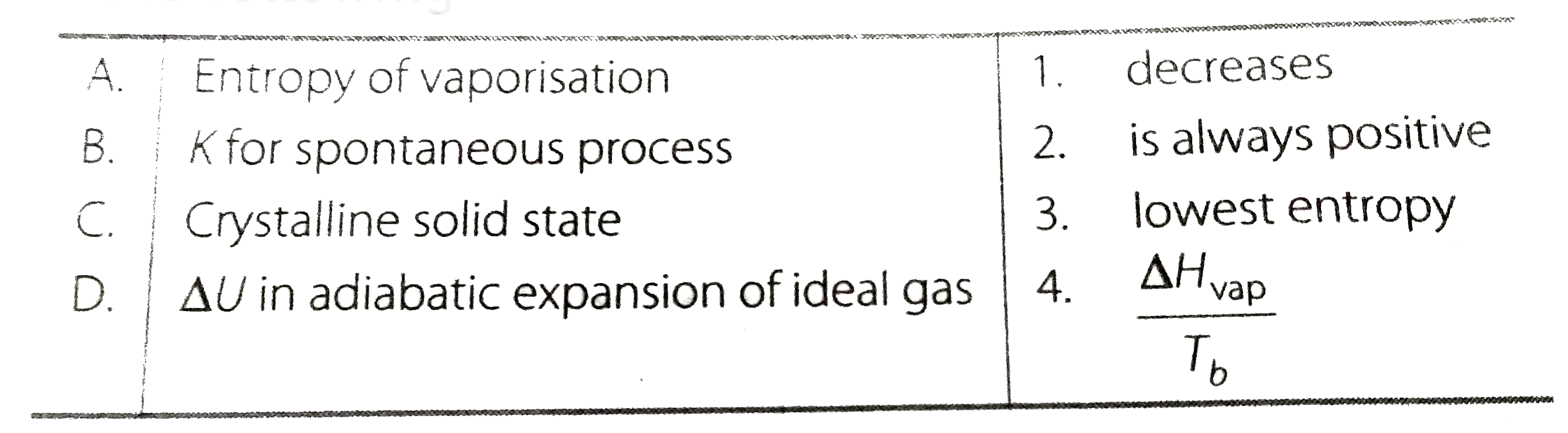# Match the following

23 views

closed
Match the followingCorrect Answer - A rarr (2, 4) B rarr (2) C. rarr (3) D. rarr(1)
A. Enthpy of vaporisation is always positive. It is equal to Delta H_("vap") //T_(b)
B. Delta_(r) G^(@) = - RT ln K
If K is positive, Delta_(r)G^(@) = negative and reaction is spontaneous.
D. During adiabatic expansion of a ideal gas, q = 0, Hence, Delta U = q + W gives Delta U = W, i.e., work done is at the cost of internal energy which decreases.